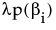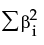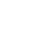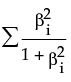The Model Launch Control Panel
After you click Go to fit a model, you can reopen the Model Launch Control Panel and change the settings to fit another model.
 • applies a penalty on the model parameters
 • uses an independent data set to assess the predictive power of the model
 • The training set is the part that estimates model parameters.
 • The validation set is the part that estimates the optimal value of the penalty, and assesses or validates the predictive ability of the model.
 • The test set is a final, independent assessment of the model’s predictive ability. The test set is available only when using a validation column.
 – If the column has three unique values, then:
 – If the column has two unique values, then only Training and Validation sets are used.
 – If the column has more than three unique values, then K-Fold validation is performed.
where x is a linear combination of the X variables.
where x is a linear combination of the X variables.Boosting is the process of building a large additive neural network model by fitting a sequence of smaller models. Each of the smaller models is fit on the scaled residuals of the previous model. The models are combined to form the larger final model. The process uses validation to assess how many component models to fit, not exceeding the specified number of models.
The learning rate must be 0 < r 1. Learning rates close to 1 result in faster convergence on a final model, but also have a higher tendency to overfit data. Use learning rates close to 1 when a small Number of Models is specified.
The penalty is, where λ is the penalty parameter, and p( ) is a function of the parameter estimates, called the penalty function. Validation is used to find the optimal value of the penalty parameter.
 Method Penalty Function Description SquaredUse this method if you think that most of your X variables are contributing to the predictive ability of the model. AbsoluteUse either of these methods if you have a large number of X variables, and you think that a few of them contribute more than others to the predictive ability of the model. Weight DecayNoPenalty none Does not use a penalty. You can use this option if you have a large amount of data and you want the fitting process to go quickly. However, this option can lead to models with lower predictive performance than models that use a penalty.

Help created on 9/19/2017